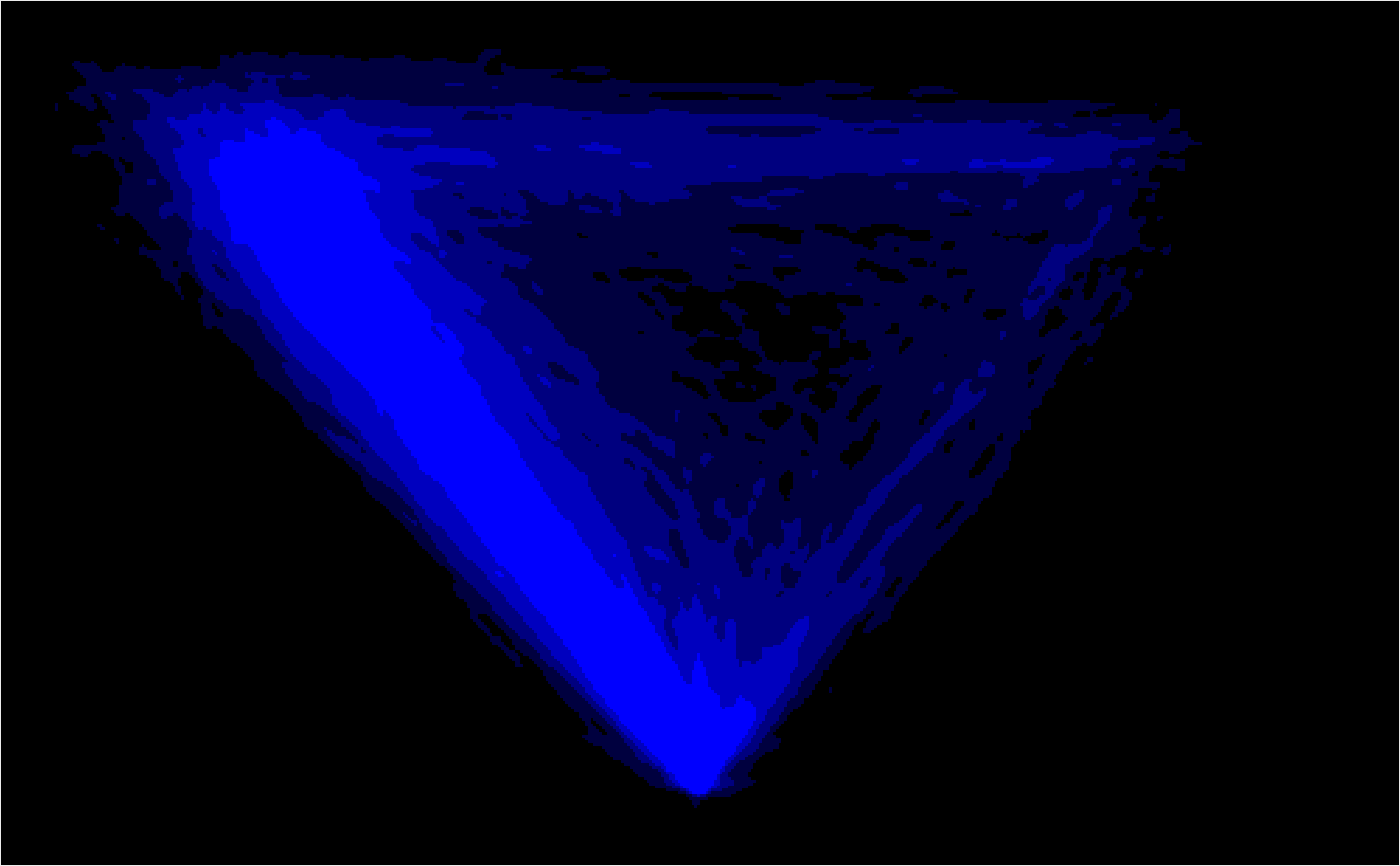mt_heatmap_ggplot plots high resolution raw trajectory maps. Note that this function has beta status.

mt_heatmap_ggplot(
data,
use = "trajectories",
dimensions = c("xpos", "ypos"),
use2 = "data",
facet_row = NULL,
facet_col = NULL,
...
)

## Arguments

data

a mousetrap data object created using one of the mt_import functions (see mt_example for details). Alternatively, a trajectory array can be provided directly (in this case use will be ignored).

use

a character string specifying which trajectory data should be used.

dimensions

a character vector specifying the trajectory variables used to create the heatmap. The first two entries are used as x and y-coordinates, the third, if provided, will be added as color information.

use2

an optional character string specifying where the data that contain the variables used for faceting can be found (in case these arguments are specified). Defaults to "data" as data[["data"]] usually contains all non mouse-tracking trial data.

facet_row

an optional character string specifying a variable in data[[use2]] that should be used for (row-wise) faceting.

facet_col

an optional character string specifying a variable in data[[use2]] that should be used for (column-wise) faceting.

...

arguments passed to mt_heatmap_raw.

## Details

mt_heatmap_ggplot wraps mt_heatmap_raw and returns a ggplot object containing the plot. In contrast to mt_heatmap_plot plots created by mt_heatmap_ggplot can be extended using ggplot's + operator. For further details on how the trajectory heatmaps are constructed, see mt_heatmap_raw.

mt_heatmap for plotting a trajectory heatmap using base plots.

mt_diffmap for plotting trajectory difference-heatmaps.

## Author

Pascal J. Kieslich

Felix Henninger

Dirk U. Wulff

## Examples

mt_heatmap_ggplot(KH2017, xres=500, n_shades=5, mean_image=0.2)
#> spatializing trajectories
#> calculate image
#> smooth image
#> enhance image by 26.5
#> Warning: Removed 1612 rows containing missing values (geom_tile()).